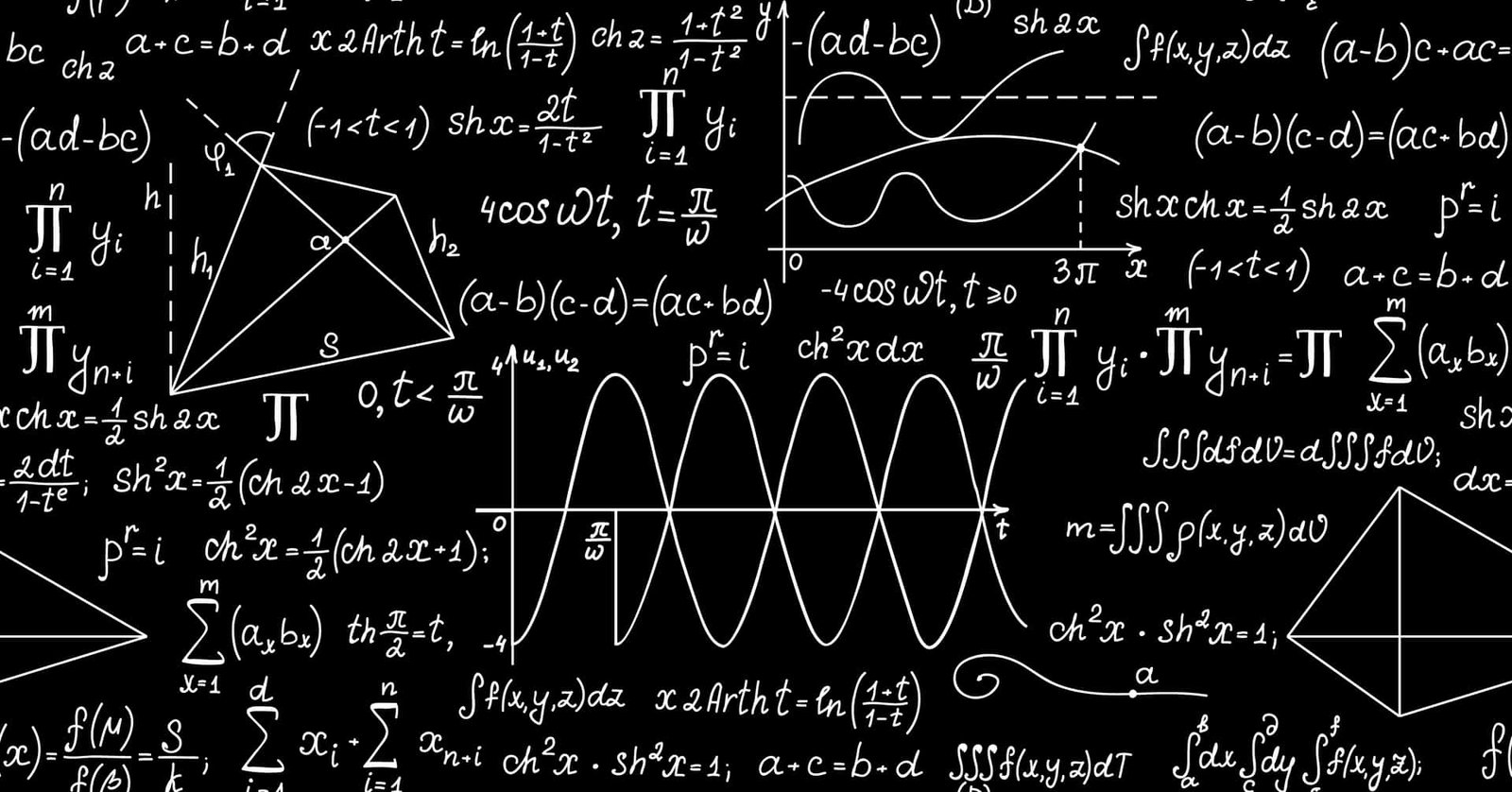Featured

### Mathematical Methods Unit 1/2

Loav Tuitions' most popular course. With plentiful resources and assistance, this course is perfect for anyone looking for that upper edge in their Math VCE journey. Whether if its to supplement your learning, to catch up or to get ahead; this course is for you! Here at Loav Tuitions, we boast astounding online support and professionally made worksheets, guides and resources as well as onsite learning to make sure you are set and sailing.

## Coverage

Unit One

AOS1 Functions, Relations and Graphs

• functions and function notation, domain, co-domain and range, representation of a function by rule, graph and table, inverse functions and their graphs
• power functions
• polynomial functions

AOS2 Algebra, Number and Structure

• symbolic notation
• transformations
• families of functions

AOS3 Calculus
• average rate of change and instantaneous rate of change.
• interpretation of graphs of empirical data with respect to rate of change such as temperature or pollution levels over time, motion graphs and the height of water in containers of different shapes that are being filled at a constant rate, with informal consideration of continuity and smoothness
• use of gradient of a tangent at a point on the graph of a function to describe and measure instantaneous rate of change of the function, including consideration of where the rate of change is positive, negative or zero, and the relationship of the gradient function to features of the graph of the original function.

AOS4 Data analysis, probability and statistics
• random experiments, sample spaces, outcomes, elementary and compound events, random variables and the distribution of results of experiments
• simulation using simple random generators such as coins, dice, spinners and pseudo-random generators using technology, and the display and interpretation of results, including informal consideration of proportions in samples
• counting methods (permutations and combinations)

Unit Two
AOS1 Functions, relations and graphs
• circular functions
• logarithmic functions and their inverse
• exponential functions and their inverse

AOS2 Algebra, Number and Structure
• log laws
• exponent Laws
• newton's Method Algorithm
• transformations

AOS3 Calculus
• limits
• first principles
• power rule
• chain rule
• product rule
• quotient rule
• special derivatives
• integration
• local maxima, minima, points of inflection
• graphical analysis
• kinematics

AOS4 Data analysis, probability, statistics
• elementary and compound events
• addition rule, mutually exclusive events
• conditional probability
• independent events
• replacement and without replacement problems